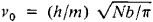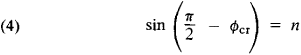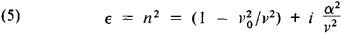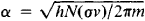# Neutron Optics

## Neutron optics

The general class of experiments designed to emphasize the wavelike character of neutrons. Like all elementary particles, neutrons can be made to display wavelike, as well as particlelike, behavior. They can be reflected and refracted, and they can scatter, diffract, and interfere, like light or any other type of wave. Many classical optical effects, such as Fresnel diffraction, have been performed with neutrons, including even those involving the construction of Fresnel zone plates. See Diffraction, Interference of waves, Reflection of electromagnetic radiation, Refraction of waves, Scattering of electromagnetic radiation, Wave (physics)

The typical energy of a neutron produced by a moderated nuclear reactor is about 0.02 eV, which is approximately equal to the kinetic energy of a particle at about room temperature (80°F or 300 K), and which corresponds to a wavelength of about 10-10 m. This is also the typical spacing of atoms in a crystal, so that solids form natural diffraction gratings for the scattering of neutrons, and much information about crystal structure can be obtained in this way. However, the wavelike properties of neutrons have been confirmed over a vast energy range from 10-7 eV to over 100 MeV. See Neutron diffraction

Neutrons, being uncharged, can be made to interfere over large spatial distances, since they are relatively unaffected by the stray fields in the laboratory that deflect charged particles. This property has been exploited by using the neutron interferometer. This device is made possible by the ability to grow essentially perfect crystals of up to 4 in. (10 cm). The typical interferometer is made from a single perfect crystal cut so that three parallel “ears” are presented to the neutron beam. This allows the incident beam to be split and subsequently recombined coherently. See Coherence, Interferometry, Single crystal

One of the most significant experiments performed with the interferometer involved rotating the interferometer about the incident beam so that one neutron path was higher than the other, creating a minute gravitational potential difference (of 10-9 eV) between the paths. This was sufficient to cause a path difference of 20 or so wavelengths between the beams. This remains the only type of experiment that has ever seen a quantum-mechanical interference effect due to gravity. It also verifies the extension of the equivalence principle to quantum theory (although in a form more subtle than its classical counterpart). See Gravitation, Relativity

Many noninterferometer experiments have also been done with neutrons. In one experiment, resonances were produced in transmitting ultracold neutrons (energy about 10-7 eV) through several sheets of material. This is theoretically similar to seeing the few lowest states in a square-well potential in the Schrödinger equation. See Neutron, Quantum mechanics

McGraw-Hill Concise Encyclopedia of Physics. © 2002 by The McGraw-Hill Companies, Inc.
The following article is from The Great Soviet Encyclopedia (1979). It might be outdated or ideologically biased.

## Neutron Optics

a branch of neutron physics that deals with a number of phenomena that have optical analogues and that arise during the interaction of neutron beams with matter or with fields (magnetic, gravitational). These phenomena are characteristic of slow neutrons and include the refraction and reflection of neutron beams at the boundary between two media, the total reflection of a neutron beam from an interface (which is observed under certain conditions), the diffraction of neutrons by individual heterogeneities in a medium (small-angle neutron scattering), and the diffraction of neutrons by periodic structures. The polarization of neutrons, which, in the first approximation, may be compared with the circular polarization of light, arises for certain substances upon reflection and refraction. The inelastic scattering of neutrons in gases, liquids, and solids is analogous to the Raman effect.

In a number of phenomena in neutron optics, the wave properties of neutrons predominate. The neutron wavelength λ is defined by the neutron mass m = 1.67 × 10-24 g and velocity v:

(1) λ = h/mv

where h is Planck’s constant. The mean velocity of thermal neutrons is ν = 2.2 × 105 cm/sec. For such neutrons, the wavelength is λ = 1.8 × 10-8 cm; that is, the wavelength is of the same order as the X-ray wavelength. The wavelengths of the slowest, or ultracold, neutrons (see below) are the same as the wavelengths of ultraviolet and visible light. The analogy between neutron beams and electromagnetic waves is also underscored by the fact that neutrons, like photons, do not have an electric charge. At the same time, neutron waves and electromagnetic waves are different in nature. Photons interact with the electron shell of the atom, whereas neutrons interact chiefly with atomic nuclei. The neutron has a rest mass, which makes it possible to use nonoptical methods for neutron studies. The presence of a magnetic moment for the neutron accounts for the magnetic interaction of neutrons with magnetic materials and magnetic fields. Photons do not interact in this manner.

The development of neutron optics began in the 1940’s, after the appearance of nuclear reactors. E. Fermi introduced the concept of the refractive index n to describe the interaction of neutrons with condensed media. When neutrons pass through a medium, they are scattered by atomic nuclei. In wave terminology, this means that an incident neutron wave produces secondary waves, and the coherent combination of these secondary waves determines the refracted and reflected waves. As a result of the interaction of neutrons with nuclei, there is a change in the velocity and, consequently, in the wavelength λ1 of neutrons in a medium, as compared with the wavelength λ in a vacuum. Under ordinary conditions, when the absorption of neutrons over a path of the order of λ1 can be neglected (just as in optics), we have n = λ/λ1. It follows from the de Broglie relation that n = λ/λ1=v1/v.

If U is the interaction potential between neutrons and nuclei, averaged over the volume of the medium, then a neutron should perform work when it strikes the medium. The kinetic energy of the neutron in the medium decreases from its initial value ε0 = mv2/2 to the value ℰ1 = ℰ – U. When U > 0, the neutron velocityin the medium decreases (ν1 < ν); in this case, we also have λ1 > λ and n < 1. When U < 0, the velocity increases and n > 1. If a quantity analogous to the dielectric constant (∊ = n2) is introduced for neutrons, then ∊ = λ212 = v12/v2 = ℰ1/ℰ.. The potential is U = h2Nb/2πm, from which

(2) ∊ = n2 = 1 - h2Nb/πm2v2

Here, b is the coherent scattering length for the scattering of neutrons by nuclei and N is the number of nuclei per unit volume of the medium. For most substances, b > 0, and equation (2) may be put in the form

(3) ∊ = n2 = 1 - v02/v2

whereNeutrons with velocity ν < vo have energy ε0 > < U; for such neutrons, n2 < 0, that is, the refractive index is imaginary. Such neutrons cannot overcome the repulsive forces of the medium and are completely reflected from the surface of the medium. They are called ultracold neutrons. For metals, vo is about several m/sec (for example, for copper, vo = 5.7 m/sec).

The velocity of thermal neutrons is several hundred times greater than the velocity of ultracold neutrons, and n is close to unity (1 —n ≈ 10-5). When a beam of thermal neutrons is incident upon the surface of a dense substance at a glancing angle, the beam undergoes total reflection analogous to the total internal reflection of light. This happens at glancing angles ɸ < Φcr, that is, at angles of incidence θ ≥ θcr = (π/2) —ɸcr. The critical angle is determined from the conditionFor example, for copper, ɸcr = 9.5’. It can be shown that the condition of total reflection (4) is equivalent to the requirement ν = ≤ νo, where vz. is the component of the neutron’s velocity that is normal to the reflecting surface. The velocity of cold neutrons is several times less than the velocity of thermal neutrons, and the angle Φcr, is correspondingly greater.

Total reflection is used to transport, with minimal losses, thermal and cold neutrons from a nuclear reactor to the experimental facilities (distances of about 100 m). This is accomplished by means of mirror neutron guides—evacuated tubes whose inner surface reflects neutrons. Mirror neutron guides are made of copper or glass and may or may not have a metal coating.

The reflection coefficient of neutrons is actually always somewhat less than unity because nuclei not only scatter neutrons but also absorb them. Taking absorption into account leads to a refinement of equation (3):whereHere, σ is the effective cross section of all processes that lead to attenuation of the neutron beam. The sum of the capture cross section and inelastic-scattering cross section is significant for cold and ultracold neutrons and is inversely proportional to the velocity ν. The product σv is therefore independent of ν. This means that for neutrons, just as in optics, ∊ and n are complex quantities: ∊ = ∊’ + i∊” and n = η” + iη’. For ultracold neutrons, the real part is ∊, that is, ∊’ < 0 and n” > n’. This is characteristic of metals in the case of light, and the reflection of ultracold neutrons from many substances is analogous to the reflection of light from metals with an extremely high reflectance (seeMETAL OPTICS). If b < 0, then a plus sign precedes the term νo22 in equation (5) and ∊ > 1 (the dielectric constant increases with decreasing ν). Such substances reflect and refract very slow neutrons, just as dielectrics reflect and refract light.

Equation (2) can be easily generalized to the case in which a magnetic field is present in the medium if we add, to the energy U of the interaction of neutrons with the medium, the energy of magnetic interaction ± μB, where μ is the magnetic moment of the neutron and B is the magnetic induction; here, the ± sign refers to the two possible orientations of the neutron’s magnetic moment with respect to the vector B, that is, the two polarizations of the neutron beam. The generalized equation is

(6) n2 = 1 - h2Nb/πm2v2 ± 2μB/mν2

By selecting the material for the reflecting mirror, the magnetic field, and the glancing angle, it is possible to ensure that the neutrons of one of the two polarizations undergo total reflection, whereas neutrons of the other polarization do not. A device making use of this principle is used to produce beams of polarized neutrons and to determine the degree of polarization of the beam.

A number of devices that are used both in experiments and in the solution of practical problems are based on the principles of neutron optics. These include neutron mirrors, straight and curved neutron guides with total internal reflection, neutron crystal monochromators, mirror and crystal neutron polarizers and analyzers, devices for focusing neutron beams, refracting prisms, and neutron interferometers. Neutron diffraction is extensively used to investigate submicroscopic properties of matter: atomic-crystal structure, vibrations of the crystal lattice, magnetic structure, and the dynamics of the crystal lattice.

### REFERENCES

Fermi, E. Lektsii po atomnoi fizike. Moscow, 1952. (Translated from English.)
Hughes, D. J. Neitronnaia optika. Moscow, 1955. (Translated from English.)
Gurevich, I. I., and L. V. Tarasov. Fizika neitronov nizkikh energii. Moscow, 1965.
Frank, I. M. “Nekotorye novye aspekty neitronnoi optiki.” Priroda, 1972, no. 9.

IU. M. OSTANEVICH and I. M. FRANK

## neutron optics

[′nü‚trän ′äp·tiks]
(physics)
The study of certain phenomena, for example, crystal diffraction, in which the wave character of neutrons dominates and leads to behavior similar to that of light.
McGraw-Hill Dictionary of Scientific & Technical Terms, 6E, Copyright © 2003 by The McGraw-Hill Companies, Inc.
Mentioned in ?
References in periodicals archive ?
They highlight new results and developments in such topics as neutron electric dipole moment searches, neutron optics and interferometry, Standard Model tests using neutron beta decay, neutron facilities, neutron polarimetry, and nucleon-nucleon interactions.
Bowman, Los Alamos National Laboratory Neutron Optics II -- Chair: David Jacobson, National Institute of Standards and Technology 2:00-2:15 Experimental test of Laue diffraction method of a search for neutron EDM V.
In my view, the PNC neutron optics experiments are most beautiful manifestations of parity-violation properties of ordinary matter.
Monte Carlo simulations are being performed to design and characterize the neutron optics components for the two fundamental neutron physics beamlines at the Spallation Neutron Source.
Key words: McStas; neutron optics; neutron sources.
Hughes, Neutron Optics, Interscience Publishers, Inc., New York (1954) Secs.
Sears, Fundamental Aspects of Neutron Optics, Phys.
Key words: neutron interferometry; neutron optics; Nowak correction; refractive index; scattering length.
The basis of neutron optics is the Schrodinger equation for the coherent interaction of the neutron with matter using an optical potential.
Valsky, Neutron optics P-violation effects near p-wave resonance, Physica B 267-268, 289-293 (1999).
Glinka (1975-present) Small-angle neutron scattering, mesoporous materials, neutron optics and scattering instrumentation.

Site: Follow: Share:
Open / Close